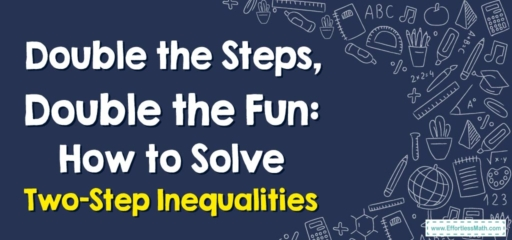# Double the Steps, Double the Fun: How to Solve Two-Step Inequalities

Today we're diving deeper into the realm of inequalities. Specifically, we're going to learn how to solve two-step inequalities. As the name implies, these inequalities usually require at least two steps to solve and find the range of possible solutions.## 1. Understanding Two-Step Inequalities

A two-step inequality is an inequality that requires two operations, such as addition/subtraction and multiplication/division, to solve. A common example could be $$2x + 3 > 7$$.

## 2. Solving Two-Step Inequalities

The process of solving two-step inequalities is very similar to solving two-step equations, but there’s an important twist when dealing with multiplication or division by a negative number.

## Step-By-Step Guide to Solving Two-Step Inequalities

Let’s dive into the step-by-step process of solving two-step inequalities.

### Step 1: Simplify If Necessary

First, simplify the inequality if possible. This might involve distributing a number or combining like terms.

### Step 2: Use Inverse Operations

Use inverse operations to get the variable by itself. Start by undoing any addition or subtraction, then undoing multiplication or division. Remember, if you multiply or divide by a negative number, the inequality symbol must be flipped!

### Step 3: Write and Check Your Solution

Write your solution as an inequality. Then, pick a number within your solution range and check if it makes the original inequality true.

For example, let’s solve the inequality $$2x + 3 > 7$$:

1. Subtract $$3$$ from both sides: $$2x > 4$$.
2. Divide both sides by $$2: x > 2$$.

So, the solution to $$2x + 3 > 7$$ is $$x > 2$$.

Solving two-step inequalities might seem challenging at first, but with practice, it becomes more intuitive. Remember, the key to mastering this is understanding each step and practicing consistently.

Stay curious and keep practicing, and you’ll see your understanding of inequalities skyrocket. Until next time, happy solving!

In this blog post, we’ve introduced two-step inequalities, explained how to solve them, and provided a step-by-step guide to practicing this important skill. Keep practicing, and soon you’ll be tackling these with ease!

### What people say about "Double the Steps, Double the Fun: How to Solve Two-Step Inequalities - Effortless Math: We Help Students Learn to LOVE Mathematics"?

No one replied yet.

X
30% OFF

Limited time only!

Save Over 30%

SAVE $5 It was$16.99 now it is \$11.99